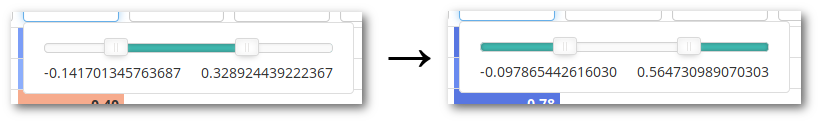While building a Shiny application with R recently, I've come across the need to invert the filterRange() function in the DT package, which provides a convenient high-level way to add DataTables to your Shiny app. As indicated by its name, this function filters a numeric column in your datatable based on a range, so as it contains only values contained within that range. What I needed was the opposite: include values outside the specified range.

The filtering is done server-side and unfortunately, no option is provided out-of-the-box to perform this inversion. One of the solutions is therefore to monkey-patch the filterRange() function in the DT R package, replacing it with a version that filters the outer range instead.

Googling for "monkey patching r" (currently) yields this blog post, which provides a more complicated though arguably cleaner solution, which introduces a new environment in the search path. My position on this is that if you're worried about cleanliness, you shouldn't be monkey-patching in the first place. Conversely, if you decide monkey-patching is acceptable in your situation, the code required should be as quick and dirty as the thought.

Of course, this is R, uncontested king of weird ways of doing anything but the most common data analysis tasks, and even some of those -- so it's never going to be as simple as Python, for instance:

import sys
sys.stdin = "foo"
# Aaand done.


But it doesn't have to be as complicated as the solution in the blog post above, either.

The solution presented here is basically taken from this mailing list post, which has the disadvantage of not containing the key term "monkey-patch", which makes it hard to find on Google. It consists in the following steps:

1. Get a handle on the relevant library's namespace with getNamespace().
2. Make the relevant binding modifiable with unlockBinding().
3. Define your custom version of the function.
4. Store it in the namespace under the original name.
5. Re-seal everything with lockBinding().

Here's the code for my specific use case with DT::filterRange():

# Monkey patch the filterRange() function in the DT package so that server-side filtering returns
# values *outside* the range instead of inside.

DT <- getNamespace("DT")
unlockBinding("filterRange", DT)

####################################################################################################
# This part of the code is deliberately kept as similar to the original as possible, in order to
# make potential updates easier. See https://github.com/rstudio/DT/blob/v0.2/R/shiny.R#L474.

# filter a numeric/date/time vector using the search string "lower ... upper"
filterRange = function(d, string) {
if (!grepl('[.]{3}', string) || length(r <- strsplit(string, '[.]{3}')[]) > 2)
stop('The range of a numeric / date / time column must be of length 2')
if (length(r) == 1) r = c(r, '')  # lower,
r = gsub('^\\s+|\\s+$', '', r) r1 = r; r2 = r if (is.numeric(d)) { r1 = as.numeric(r1); r2 = as.numeric(r2) } else if (inherits(d, 'Date')) { if (r1 != '') r1 = as.Date(r1) if (r2 != '') r2 = as.Date(r2) } else { if (r1 != '') r1 = as.POSIXct(r1, tz = 'GMT', '%Y-%m-%dT%H:%M:%S') if (r2 != '') r2 = as.POSIXct(r2, tz = 'GMT', '%Y-%m-%dT%H:%M:%S') } if (r == '') return(d <= r2) if (r == '') return(d >= r1) d <= r1 | d >= r2 } # End pastiche of original DT code. #################################################################################################### DT$filterRange <- filterRange
lockBinding("filterRange", DT)


EDIT, 2021-04-13: As Vincent Wolowski points out in the comments, you can also call the higher-level function assignInNamespace, which does the unlock/relock dance for you behind the scenes: assignInNamespace('filterRange', filterRange, 'DT').

The last piece of the puzzle concerns UX: the user should understand that the filter applies to the outer range, not the inner one. Visually:This is easily achieved with a few lines of CSS:

#datatable-id .noUi-background {
background: #3FB8AF;
box-shadow: inset 0 0 3px rgba(51,51,51,.45);
transition: background 450ms;
}

#datatable-id .noUi-connect {
background: #FAFAFA;
box-shadow: inset 0 1px 1px #f0f0f0;
}


In conclusion, monkey-patching is rarely the most elegant, debuggable and maintainable solution to a problem you're having. More often, it's actually the least elegant (etc.) one. But every once in a while, it's the simplest one, the one with the best hassle/reward ratio (until it comes back to bite you once your codebase has grown or assumptions about the monkey-patched code have changed). At any rate, if you need to resort to it, it's nice to have a quick, googlable how-to, hence this post.